Looking for revision notes that are specific to the exam board you are studying? If so, click the links below to view our condensed, easy-to-understand revision notes for each exam board, practice exam question booklets, mindmap visual aids, interactive quizzes, PowerPoint presentations and a library of past papers directly from the exam boards.

# Atomic Symbols - The Modern Periodic Table

Every atom is made up of three crucial components – The neutral neutron, the positively charged proton and the negatively charged electron. Among these, the number of protons gives the identity to an element while the number of neutrons gives the identity to the isotopes of an element and the number of electrons gives identity to the elemental or ionic state of the concerned element.

In a neutral atom, the number of protons equals the number of electrons, although, the number of neutrons may differ according to the isotope. As a result, it becomes a necessity to provide a system of representation that can distinguish between the isotopes as well as different elements simultaneously.

An atomic symbol consists of three parts represented as:The mass number is the sum of the number of neutrons and protons.

Atomic number is the number of protons.

The electrical charge represents the net gain (for anions) or loss (for cations) of electrons.

X represents the alphabetical symbol.

Some of the important points that need to be remembered are:

1. The symbol may be written without the atomic number Z, as it is characteristic of each element and no two elements have the same alphabetical symbol X.
2. The symbol X can either contain a single alphabet, represented with CAPITAL LETTERS or two alphabets, represented with a CAPITAL LETTER followed by a “small letter”.
3. The mass number must be included in the symbol to identify the isotope.
4. The representation of the element without mass number usually denotes the collection of the atoms of an element in the order of its natural abundance.
5. Electrical charge is not needed for representation of a neutral atom since there is neither excess nor lack of electrons in it. However, it is needed for an ion.
6. The periodic table includes the atomic symbols with the atomic mass/ weight, instead of the mass number.Utility of the Atomic symbols

1. The atomic symbols are useful in determining the number of protons, neutrons, and electrons.
2. The atomic symbols are useful in identifying the components of a compound.
3. The atomic symbols are useful in identifying the group and the period to which the element belongs.
4. The atomic symbols are useful in determining the electronic configuration.

The number of protons, neutrons, and electrons can be easily obtained by the following method.

Let’s look at the following: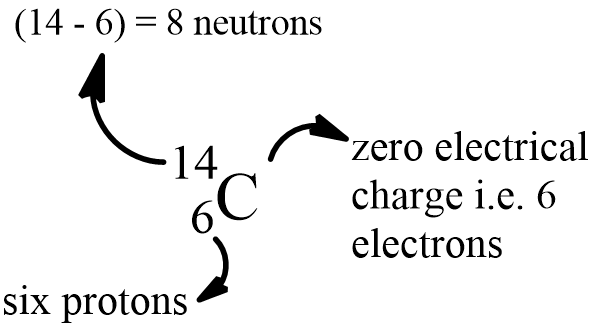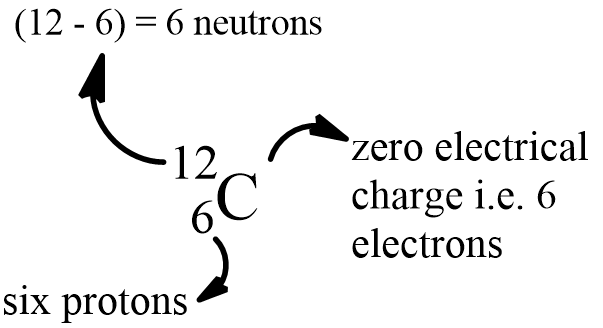The carbon-12 has 6 protons, six neutrons, and 6 electrons since the number of protons & electrons must be equal for it to be neutral. Carbon – 14, on the other hand, has 8 neutrons.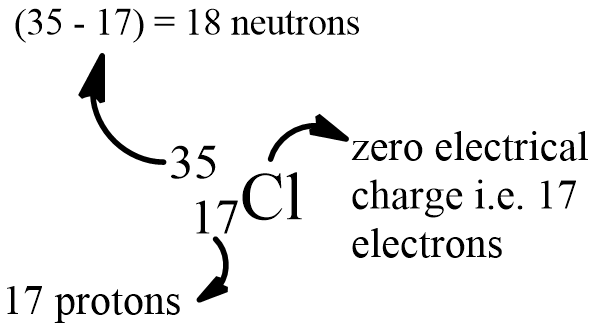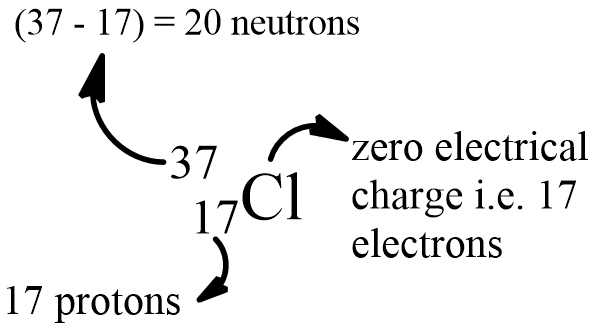Both isotopes of chlorine contain an equal number of protons and electrons.

While calculating the number of electrons in an ion, we should subtract the positive charge (for cation) from the number of protons and add the negative charge (for anion) to the number of protons.

Chloride ion (formed from Cl – 37)Magnesium ion (formed from Mg – 24)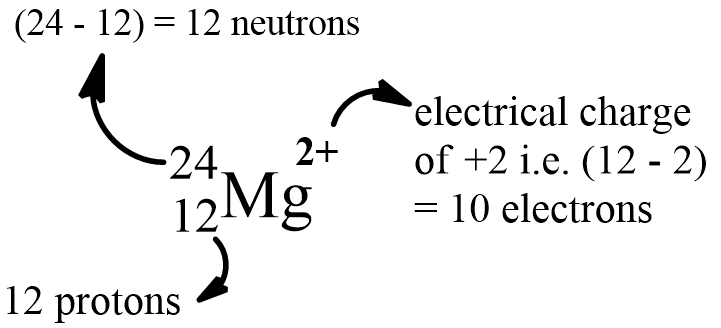The atomic symbols are hence the easiest tools to quickly obtain the essential information about an element.

References: